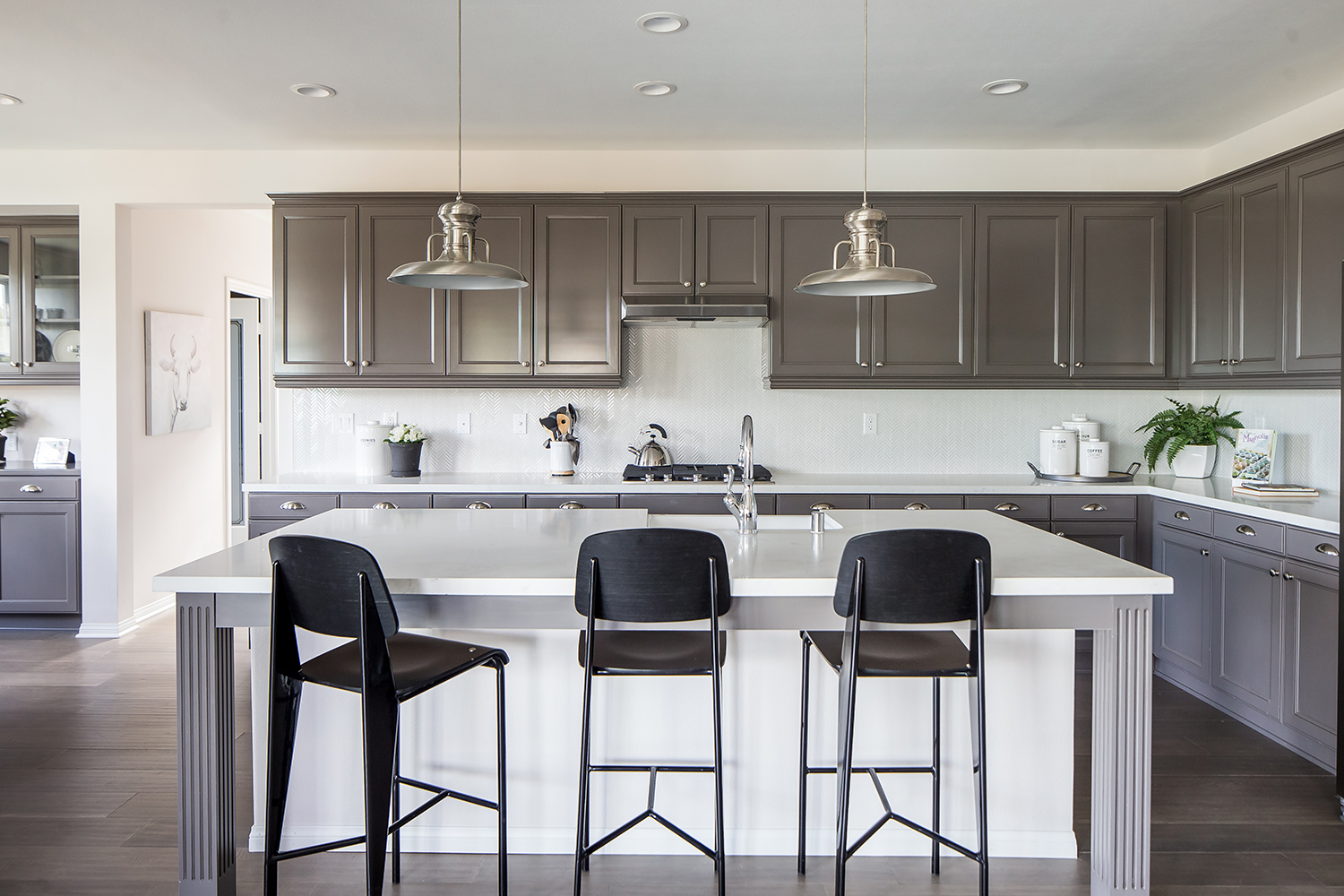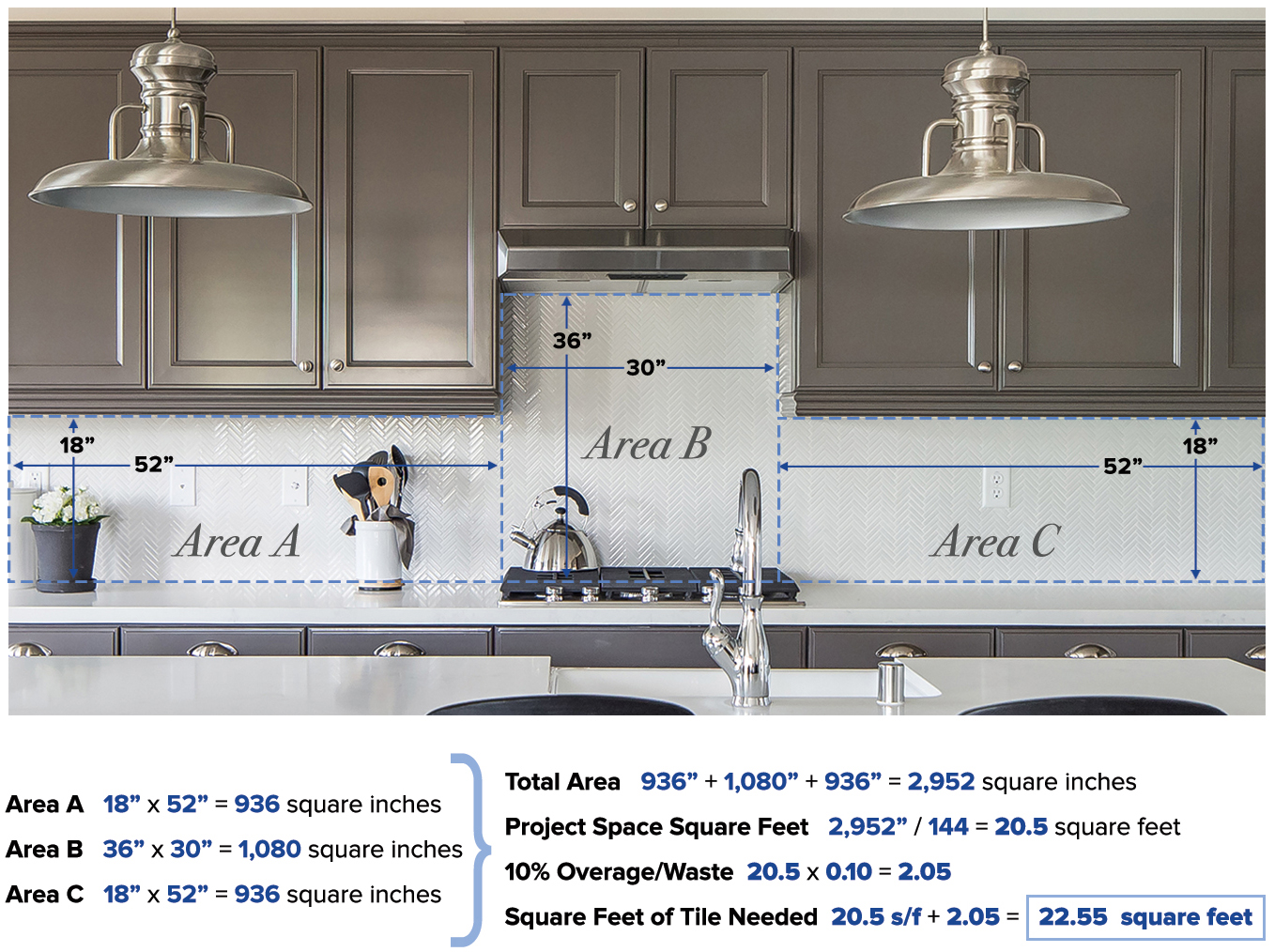Do You Measure Up? | Bedrosians Tile & Stone

# Do You Measure Up?

Whether you’re planning on installing your own tile floor or backsplash, or simply trying to figure out how much material you'll need for your project and budget, read on to learn how to measure the square footage of your space.###### 90° Herringbone Mosaic in White (Image credit: The Farm at Ventura)

With a measuring tape and simple math, you can estimate how much tile you need and how much the project is going to cost you.

Simple things you'll need:Measuring tapeSomething to write withSomething to write onCalculator (or if you're extra smart, just your head)Step 1) Break down the area into manageable parts. Split up the space into even squares or rectangles. To find the square footage, or the area, of the entire space, you'll need to find the square inches of the individual spaces.

See Areas A, B and C

Step 2) Find the square inches (area). Multiply the length of each space by the width of the each space.

A: 18"x52" = 936 in2
B: 36"x30" = 1,080 in2
C: 18"x52" = 936 in2

Step 3) Calculate the total area in total inches. Add the square inches of your spaces together.

936  +  1,080  +  936  =  2,952 in2

Step 4) Find the total area square feet. Take the total square inches and divide that by 144.

2,952  /  144  =  20.5 sf

Step 5) Don't forget the overage/waste - add on 10%. Never buy just the amount of tile you need, since you always need a bit extra for cuts, waste, breaks, and mistakes. To get the overage amount, multiply the square footage of the space you're tiling by 10%.

20.5  x  0.10  =  2.05

Step 6) How much tile should I buy? To calculate how much to order, add the overage to the total square of the room.

20.5 sf  +  2.05  =  22.55 square feet to buy!

Helpful tip 1 about overage: Another reason to buy extra tile is if the style or color is discontinued and you need to replace a broken tile or two, you will need extras on hand to make the repairs. If you don't, then in order to fix it, you will have to replace the entire floor, backsplash, wall area. Spend a little bit more up front to save money (and headaches) in the long run.

Helpful tip 2 about overage: The larger the tile or the more complicated the pattern or mosaic, the more waste you'll have.  So work with your Bedrosians sales representative to figure exactly how much tile is needed to complete your project.

## Q & A

### What if the wall isn't a simple rectangle? What if the room isn't square?

If the space you want to tile is an unusual shape, draw a picture of the floor plan on a piece of paper, then separate the room into squares or rectangles (like in the diagram above). Then measure each squared-off section separately, convert inches to feet, divide by 12, multiply the length and width of each space, then add all these numbers together to find the total square footage.

### How many square feet of tile comes in a box?

It really depends on the tile your purchase. Bedrosians sells it field tile in box quantities and mosaics by the square foot.

### How many boxes of tile will I need?

Divide the total square footage you need by the amount included in the box of tile. If you need 165 square feet, and each box holds 10, then divide 10 into 165: You'll need 16.5 boxes. So buy 17! Or 18, just to be sure. Your Bedrosians sales representative can help you figure out how much tile comes comes in a box, as it pertains to your specific order.

Want to see more from Bedrosians?

Receive the latest info on product arrivals, trends, and design tips.